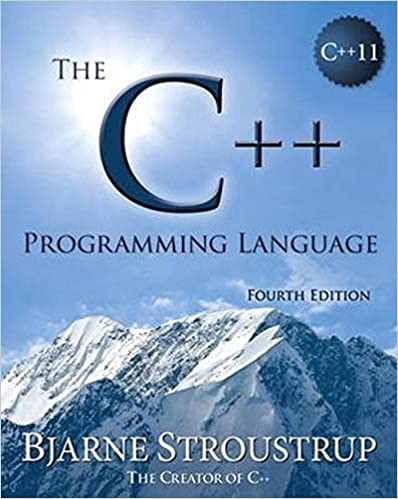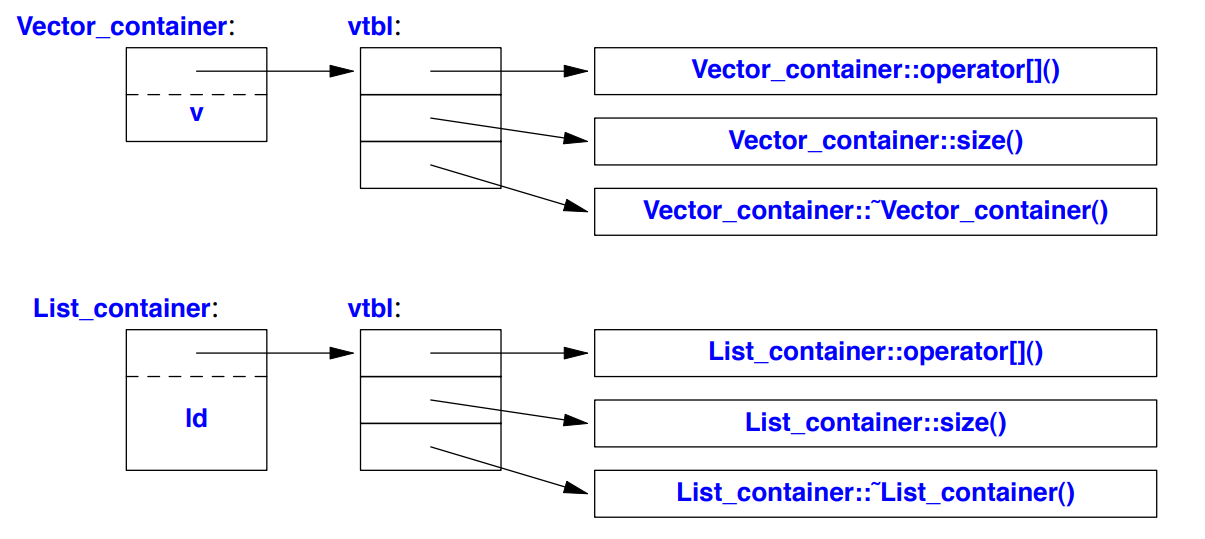# Abstraction MechanismsDon’t Panic!

## Abstract Types

### Interface

class Container {
public:
virtual double& operator[](int) = 0; // pure virtual function
virtual int size() const = 0;        // const member function
virtual ~Container() {}              // destructor
}


Above class is a pure interface to specific containers defined later. The word virtual means “may be redefined later in a class derived from this one.”

• virtual function: A function declared virtual
A class derived from Container provides an implementation for the interface(virtual functions).
• pure virtual: A function syntax with =0; that is, some class derived from Container must define the function.
• abstract class: A class with a pure virtual function; it is impossible to define an object of class type with pure virtual function. A class that provides the interface to a variety of other classes is often called a polymorphic type.

### Inheritance

class Vector_container : public Container { // Vector_container implements Container
Vector v;
public:
Vector_container(int s) : v(s) {} // constructor
~Vector_container() {}            // destructor

double& operator[](int i) { return v[i]; }
int size() const { return v.size(); }
}

• Class Vector_container(subclass) “is derived from” / “is a subtype of” class Container(superclass).
• Class Container is “a base of” class Vector_container.

When superclass’s virtual function is called, proper subclass’s override function must be called. The object must contain information to allow it to select the right function to call at run time. Each class with virtual functions has its own virtual function table identifying its virtual functions.The implementation of the caller needs only to know the location of the pointer to the virtual function table in a Container and index used for each virtual function.

A class hierarchy offers two kinds of benefits:

• Interface inheritance: An object of a derived class can be used wherever an object of a base class is required.
That is, the base class acts as an interface for the derived class.
• Implementation inheritance: A base class provides functions or data that simplifies the implementation of derived class.

## Copy and Move

By default, objects can be copied. The default meaning of copy is memberwise copy; copy each member.

void test(Complex z1) {
Complex z2 {z1}; // copy initialization
Complex z3;
z3 = z2;         // copy assignment
// ...
}


### Copy Constructor & Copy Assignment

When a class is a resource handle; it is responsible for an object accessed through a pointer, the default memberwise copy is a disaster.
Two different objects of class might (unwillingly) share same resource.We don’t want v1 & v2 to point to the same resource. We want v1 & v2 to have own resource with same value.

Copying of an object of a class can be defined by two members: a copy constructor and a copy assignment.

class Vector {
private:
double* elem;
int sz;
public:
Vector(int s);
~vector() { delete[] elem; }

// copy constructor
Vector(const Vector& other) : elem{new double[other.sz]}, sz(other.sz) {
for (int i = 0; i < sz; i++)
elem[i] = other.elem[i];
}

// copy assignment
Vector& operator=(const vector& other) {
if (sz != other.sz) {
delete[] elem;
elem = new double[other.sz];
sz = other.sz;
}

for (int i = 0; i < sz; i++)
elem[i] = other.elem[i];
return *this;
}

// ...
}


The book used elem{new double[sz]} for the copy constructor. Which I believe, is wrong.
According to the reference, the order of member initializers in the list is irrelevant: the actual order of initialization is as follows:

1) If the constructor is for the most-derived class, virtual bases are initialized in the order in which they appear in depth-first left-to-right traversal of the base class declarations (left-to-right refers to the appearance in base-specifier lists)
2) Then, direct bases are initialized in left-to-right order as they appear in this class’s base-specifier list
3) Then, non-static data member are initialized in order of declaration in the class definition.
4) Finally, the body of the constructor is executed

I also modified copy assignment as my taste.(Bit more of a optimization)

### Move Constructor & Move Assignment

Sometimes, copying can be costly for large objects. And original object is no longer needed right after the use.

class Vector {
private:
double* elem;
int sz;
public:
// ...

// move constructor
Vector(Vector&& other) : elem(other.elem), sz(other.sz) {
// reset rvalue reference
other.elem = nullptr;
other.sz = 0;
}

// move assignment
Vector& operator=(Vector&& other) {
// free currently holding array
delete[] elem;

// "grab the elements" from rvalue reference
elem = other.elem;
sz = other.sz;

// reset rvalue reference
other.elem = nullptr;
other.sz = 0;
}

// ...
}


&& means “rvalue reference”. The word “rvalue” roughly means “something that can’t assign to”; such as an returned object from a function call. An rvalue reference is a reference to something that nobody else can assign to (and ideally not be used afterword).

A move constructor & assignment does not take const argument: they supposed to remove the value from its argument.

After a move, a moved-from object should be in a state that allows a destructor to be run.
Typically, we should also allow assignment to a moved from object.
This is something we often forget about, but very important to prevent malfunction.

### Suppressing Operations

Sometimes the best thing to do is to delete the default copy & move operations; that is, to eliminate the default definitions of functions:

class SuppressedClass {
public:
// no copy operations
SuppressedClass(const SuppressedClass&) = delete;
SuppressedClass& operator=(const SuppressedClass&) = delete;

// no move operations
SuppressedClass(SuppressedClass&&) = delete;
SuppressedClass& operator=(SuppressedClass&&) = delete;

// ...
}


Any attempt to copy or moving a SuppressedClass will be caught by the compiler.

A move operation is not implicitly generated for a class where the user has explicitly declared a destructor.

The =delete mechanism is general; that is, it can be used to suppress any operation.

## Templates

A template is a class or a function that we parameterize with a set of types or values.

template<typename T>
class Vector {
private:
T* elem;
int sz;
public:
Vector(int s);
~vector() { delete[] elem; }

// copy and move operations...

T& operator[](int i);
const T& operator[](int i);

// ...
}


The template<typename T> prefix makes T a parameter of the declaration it prefixes.

template<typename T> & template<class T> are generally interchangeable(and have same meaning), except for some special cases.

### Function Objects

Function object (or functor) is used to define objects that can be called like functions. For example:

template<typename T>
class Less_than {
const T val;
public:
Less_than(const T& v) : val(v) {}
bool operator()(const T& x) const { return x < val; } // call operator
}


The function called operator() implements the “function call”, “call”, or “application” operator ().

We can define named variables of type Less_than for some argument type:

Less_than<int> lti {42};          // lti(i) will compare i to 42 using < (i<42)
Less_than<string> lts {"Backus"}; // lts(s) will compare s to "Backus" using < (s<"Backus")


We can call such an object, just as we call a function:

void fct(int n, const string & s)
{
bool b1 = lti(n); // true if n<42
bool b2 = lts(s); // true if s<"Backus"
// ...
}


A template can be defined to accept an arbitrary number of arguments of arbitrary types.

template<typename T, typename ... Tail>